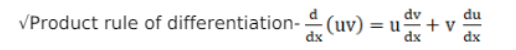Deepak Scored 45->99%ile with Bounce Back Crack Course. You can do it too!

# If the solve the problem

Question:

If $y=x+\tan x$, show that: $\cos ^{2} x \frac{d^{2} y}{d x^{2}}-2 y-2 x=0$

Solution:

Basic idea:

$\sqrt{S e c o n d}$ order derivative is nothing but derivative of derivative i.e. $\frac{\mathrm{d}^{2} \mathrm{y}}{\mathrm{dx}^{2}}=\frac{\mathrm{d}}{\mathrm{dx}}\left(\frac{\mathrm{dy}}{\mathrm{dx}}\right)$

$\sqrt{T h e}$ idea of chain rule of differentiation: If $f$ is any real-valued function which is the composition of two functions $u$ and $v$, i.e. $f=v(u(x))$. For the sake of simplicity just assume $t=u(x)$

Then $f=v(t) .$ By chain rule, we can write the derivative of $f$ w.r.t to $x$ as:

$\frac{\mathrm{df}}{\mathrm{dx}}=\frac{\mathrm{dv}}{\mathrm{dt}} \times \frac{\mathrm{dt}}{\mathrm{dx}}$Apart from these remember the derivatives of some important functions like exponential, logarithmic, trigonometric etc..

Let's solve now:

Given, $y=x+\tan x \ldots . .$ equation 1

As we have to prove: $\cos ^{2} x \frac{d^{2} y}{d x^{2}}-2 y+2 x=0$

We notice a second-order derivative in the expression to be proved so first take the step to find the second order derivative.

Let's find $\frac{d^{2} y}{d x^{2}}$

$\operatorname{As} \frac{\mathrm{d}^{2} \mathrm{y}}{\mathrm{dx}^{2}}=\frac{\mathrm{d}}{\mathrm{dx}}\left(\frac{\mathrm{dy}}{\mathrm{dx}}\right)$

So lets first find $d y / d x$ and differentiate it again.

$\therefore \frac{d y}{d x}=\frac{d}{d x}(x+\tan x)=\frac{d}{d x}(x)+\frac{d}{d x}(\tan x)\left[\because \frac{d}{d x}(\tan x)=\sec ^{2} x \& \frac{d}{d x}\left(x^{n}\right)=n x^{n-1}\right]$

$=1+\sec ^{2} x$

$\therefore \frac{d y}{d x}=1+\sec ^{2} x$

Differentiating again with respect to $x$ :

$\frac{d}{d x}\left(\frac{d y}{d x}\right)=\frac{d}{d x}\left(1+\sec ^{2} x\right)=\frac{d}{d x}(1)+\frac{d}{d x}\left(\sec ^{2} x\right)$

$\frac{\mathrm{d}^{2} \mathrm{y}}{\mathrm{dx}^{2}}=0+2 \sec \mathrm{x} \sec \mathrm{x} \tan \mathrm{x}$

[ differentiated $\sec ^{2} x$ using chain rule, let $t=\sec x$ and $z=t^{2} \therefore \frac{d z}{d x}=\frac{d z}{d t} \times \frac{d t}{d x}$ ]

$\frac{\mathrm{d}^{2} \mathrm{y}}{\mathrm{dx}^{2}}=2 \sec ^{2} \mathrm{x} \tan \mathrm{x}$

As we got an expression for the second order, as we need $\cos ^{2} x \operatorname{term} w i t h \frac{d^{2} y}{d x^{2}}$

Multiply both sides of equation 1 with $\cos ^{2} x$ :

$\therefore$ we have,

$\cos ^{2} x \frac{d^{2} y}{d x^{2}}=2 \cos ^{2} x \sec ^{2} x \tan x[\because \cos x \times \sec x=1]$

$\cos ^{2} x \frac{d^{2} y}{d x^{2}}=2 \tan x$

From equation 1:

$\tan x=y-x_{\therefore} \cos ^{2} x \frac{d^{2} y}{d x^{2}}=2(y-x)$

$\therefore \cos ^{2} x \frac{d^{2} y}{d x^{2}}-2 y+2 x=0 \ldots$ proved